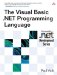# Assignment

 <  Day Day Up  >

The assignment statement assigns a value to a variable. The syntax is straightforward and should be familiar by now, given the examples used in the book so far. One thing that should be kept in mind is that, unlike some languages, such as C, the assignment operator is a statement and cannot be used within an expression ”in an expression the equals sign means equality, not assignment. For example:

` Dim x As Integer Dim y As Boolean x = 5 y = x = 5 `

In the example, the first statement assigns the value 5 to x . In the second statement, the first equals sign is assignment, while the second equals sign is equality. So the statement first calculates whether x is equal to 5 and then assigns the result ( True ) to the variable y .

## Compatibility

Previous versions of Visual Basic made a distinction between value assignment ( Let ) and reference assignment ( Set ). Let and Set assignments are no longer supported, because the .NET Framework does not allow for the distinction.

When the result of an operation is assigned to a variable that is part of the operation, compound assignment operators can be used. For example:

` Dim x As Integer = 10 x += 5    ' equivalent to x = x + 5 x *= 10   ' equivalent to x = x * 10 x \= 2    ' equivalent to x = x \ 2 `

When a compound assignment is performed, the left-hand side of the assignment (i.e., the variable being assigned to) is evaluated only once. In most cases, this makes no difference, but it does affect the situation where the variable being assigned to is an element of an array or an indexed property. In that case, the index values are evaluated only once.

` Module Test   Function a() As Integer     Console.WriteLine("Got index")     Return 0   End Function   Sub Main()     Dim x(10, 10) As Integer     x(a(), a()) += 5   End Sub End Module `

In this example, the string " Got index " will be printed only twice ”the function a will be invoked only twice, not four times. The values of each invocation will be stored and used to determine the value on the right-hand side of the assignment and the place to store the value on the left-hand side of the assignment.

 <  Day Day Up  >The Visual Basic .NET Programming Language
ISBN: 0321169514
EAN: 2147483647
Year: 2004
Pages: 173
Authors: Paul Vick

Similar book on Amazon